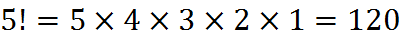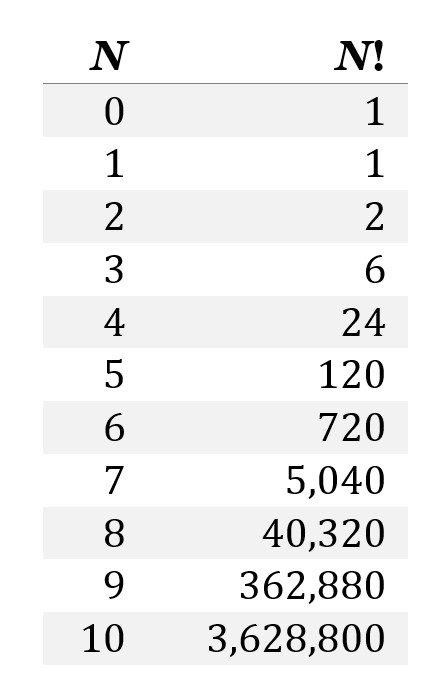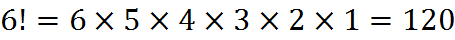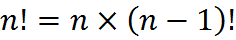# Factorials

Counting the possible ways that something can happen is a topic of some GMAT questions, and the mathematics of counting tends to involve factorials, which are labeled with an exclamation point, such as 5! or n!.

The factorial of an integer n, denoted by n!, is the product of all positive integers less than or equal to n. For example,The value of 0! is 1, by definition.As you can see, as n increases, the factorial of n increases very quickly.

Let’s look at the factorials of two adjacent numbers:You can see here that 6! includes all of the factors of 5!. In fact, the factorial of 6 is just 6 times the factorial of 5. The factorial of any number equals that number multiplied by the factorial of the number one less than it. In other words,## Practice Questions

Divisibility of a Large Number:
http://www.gmatfree.com/divisibility-of-a-large-number

Welcome! You are encouraged to register with the site and login (for free). When you register, you support the site and your question history is saved.